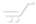# Formula operators.

Operators specify the type of calculation that is performed on the terms of a formula. Each operator can combine a specific number of formula terms (called the operands of the operator). This number ranges from one, in the case of the "not" operator, to three, in the case of the "if" operator.

NumericBase includes seven different types of operators: arithmetic, comparison, concatenation, logical, member access, the "if" operator, and the "alt" operator

Arithmetic operators.
These operators perform basic mathematical operations such as addition, subtraction, or multiplication. They produce output value of numeric type.

These operators expect input value of numeric type, if the input values are not a numeric value then they are converted automatically.

The different arithmetic operators are listed in the following table:

 Arithmetic operator Number of operands Arithmetic function Example + (plus sign) 2 Addition 5+3 - (minus sign) 2 Subtraction 9-3 - (minus sign) 1 Negation -7 * (asterisk) 2 Multiplication 2*9 / (forward slash) 2 Division 3/5
Table: The arithmetic operators.

Comparison operators
These operators perform basic comparison operations such as less than, equal, etc. They get 2 input values and produce one output value of boolean type.

The different comparison operators are listed in the following table:

 Comparison operator Meaning Example = (equal sign) Equal to 4=4 > (greater than sign) Greater than product>200 < (less than sign) Less than 34 < 45 >= (greater than or equal to sign) Greater than or equal to 45>=454 <= (less than or equal to sign) Less than or equal to 554<=545 <> (not equal to sign) Not equal to 5<>7
Table: Comparison operators.

Concatenation operator.
This operator concatenates two or more strings to produce a single string. It produces output value of text type.

This operators expects input values of text type, if the input values are not a text value then they are converted automatically.

This operator is described in the following table.

 Operator Meaning Example & (ampersand) Connects, or concatenates, two values to produce one continuous text value "key" & "board" produced "keyboard"
Table: The concatenation operator.

Logical operators.
These operators perform basic logical operations. They expect boolean values as the values of their operands and produces one boolean value as output. If the input values are not of boolean type, then they are converted automatically.

The different comparison operators are listed in the following table:

 Logical operator Number of operands meanings Example and 2 True if both input values are true 4>3 and amount<3 or 2 True if one of the input value if true 5>6 or 4<5 not 1 Reverses the value of its input value. not 4>5
Table: The logical operators.

Access operators.
Consist of the dot operator(.),the index operator([]), and the "at" operator (@). Some examples for usage:
1. Table.a
2. a
3. expanses@quorter1